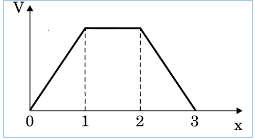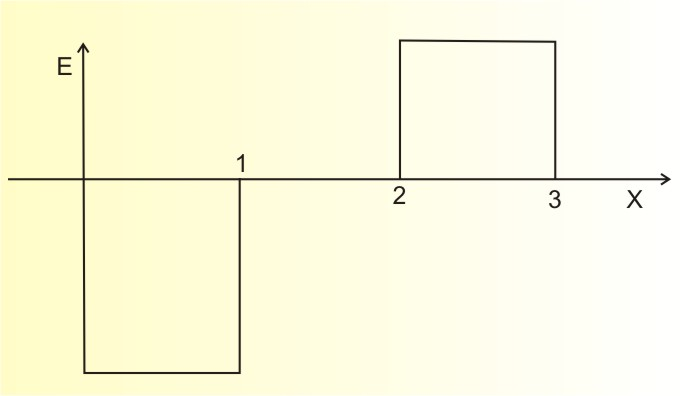# (a) When a parallel plate capacitor is connected across a dc battery, explain briefly how the capacitor gets charged. (b) A parallel plate capacitor of capacitance 'C' is charged to 'V' volt by a battery. After some time the battery is disconnected and the distance between the plates is doubled. Now a slab of dielectric constant 1<k<2 is introduced to fill the space between the plates. How will the following be affected? (i)The electric field between the plates of the capacitor.(ii) The energy stored in the capacitor.Justify your answer in each case. (c)The electric potential as a function of distance 'x' is shown in the figure. Draw a graph of the electric field E as a function of x.(a) When the capacitors are connected in parallel with a
D.C source, the charging of the capacitor:
the plates get charged in accordance with the terminals of the battery so, that the potential difference between the plates will become equal to the terminal potential of d.c battery.
(b) (i)The electric field between the plates of the parallel plate capacitor

where,  surface charge density
If the dielectric is inserted then,

where K is dielectric constant. and hence, Electric field intensity decreases to   times.
(ii)Effect on energy stored in the capacitor;
since the energy stored in the capacitor is given as;

where d=distance between two plates
Similarity:

Therefore, the energy stored between the plates increases.
(c) The graph of the electric field E as a function of x;### Preparation Products

##### JEE Main Rank Booster 2021

This course will help student to be better prepared and study in the right direction for JEE Main..

₹ 13999/- ₹ 9999/-
##### Rank Booster NEET 2021

This course will help student to be better prepared and study in the right direction for NEET..

₹ 13999/- ₹ 9999/-
##### Knockout JEE Main April 2021 (Subscription)

An exhaustive E-learning program for the complete preparation of JEE Main..

₹ 6999/- ₹ 5/-
##### Knockout NEET May 2021

An exhaustive E-learning program for the complete preparation of NEET..

₹ 22999/- ₹ 14999/-